[Next]: Research Group Numerical Mathematics and Scientific
 [Up]: Project descriptions
 [Previous]: Multiscale systems
[Contents]   [Index]

Subsections

## Homoclinic bifurcations

Collaborator: D.V. Turaev

#### Project 1: Dynamics near homoclinic tangencies

Cooperation with: S.V. Gonchenko, L.P. Shilnikov (Institute for Applied Mathematics and Cybernetics, Nizhny Novgorod, Russia)

Supported by: DFG grant no. 436 RUS, Prize of the Alexander von Humboldt Foundation

Description:

Since Poincaré's pioneering work, orbits which are homoclinic to saddle periodic ones have been one of the most attractive objects of study in the theory of dynamical systems. The main reason to study homoclinic orbits is that their presence implies complicated dynamics. It is well known that in any neighborhood of a transverse homoclinic orbit there exist infinitely many saddle periodic orbits and a continuum of non-trivial hyperbolic recurrent orbits. If the system has a non-transverse homoclinic orbit, that is, in the case of homoclinic tangency, then in any neighborhood of the given system there exist infinitely many Newhouse regions where systems with homoclinic tangencies are dense.

Since Newhouse regions exist near any system with a homoclinic tangency, it follows that they can be found in the space of parameters of any dynamical model demonstrating chaotic behavior in absence of uniform hyperbolicity, in particular in such popular examples as Hénon map, Chua circuit, Lorenz model, etc. Moreover, as numerics shows, the Newhouse regions can occupy quite large portions of the parameter space. Therefore, the problem of understanding the nature of the orbit structure for systems from Newhouse regions is quite challenging.

We have found that the dynamics of systems from the Newhouse regions is extremely rich and unusual (see Project 2). As it was shown in , a description of the dynamics of such systems requires an infinite set of invariants which means that any attempt to give a complete description of the dynamics and bifurcations in the Newhouse regions will fail. Therefore, we have to restrict the analysis to some particular details or to some most general features only.

As the most important of such general properties of systems in the Newhouse regions, we select the coexistence of many periodic orbits of different stability types (i.e., with different numbers of positive/negative Lyapunov exponents). It has been demonstrated in  that stable periodic orbits can coexist with saddle ones near a homoclinic tangency in three-dimensional systems. The questions which conditions provide the same phenomenon in the multidimensional case and which conditions could lead to the coexistence of saddles of different types within the regions of chaotic dynamics were raised by many authors. In the present work  we give a complete answer to these questions for systems from the Newhouse regions.

Consequently, following , we classify codimension-1 homoclinic tangencies by the value of the so-called effective dimension de. It is an integer which can be effectively computed for any given system with a homoclinic tangency. We have proven the following result.

Theorem 1. In the Newhouse regions the values of parameters are dense (and form a residual set) for which the system has infinitely many coexisting hyperbolic periodic orbits of (de+1) different stability types.

We describe exactly the types of these periodic orbits for different types of the Newhouse regions and show that orbits of other stability types cannot exist here. So, the question for the maximal and minimal number of positive Lyapunov exponents of the orbits of systems under consideration, as well as the question for the coexistence of orbits with different numbers of positive Lyapunov exponents, has been solved completely. In particular, we obtain necessary and sufficient conditions for the denseness of systems with infinitely many stable periodic orbits in the Newhouse regions.

We also address the question for the coexistence of infinitely many non-trivial attractors. We have proven the following result.

Theorem 2. In the case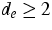, the values of parameters in the Newhouse regions are dense and form a residual set for which the system has infinitely many coexisting stable invariant tori.

The problem of the coexistence of infinitely many strange attractors in the case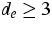was also discussed in .

#### Project 2: Richness of chaotic dynamics in non-hyperbolic systems

Description:

It has been proved in  that systems with homoclinic tangencies of arbitrarily high order are dense in the Newhouse regions, as well as systems having degenerate periodic orbits with all Lyapunov coefficients equal to zero. This implies that bifurcations of such systems are extremely involved and rich. Thus, the question arises: Can we estimate in exact terms how rich these bifurcations are? In  we give a definite answer for the example of two-dimensional area-preserving maps. It is shown that we deal here with the ultimate richness: Every possible dynamics can be obtained by a small perturbation of any system from a Newhouse region.

To be more precise, let f be a Cr-smooth area-preserving map of R2. Define the dynamical conjugacy class of f as the set of all maps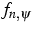of a unit disc U1 into R2 obtained by the rule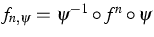, where n is an integer, fn is the n-th iteration of f, and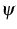is an arbitrary Cr-smooth map of U1 into R2 with a constant Jacobian (hence, by construction, all the mapsin the class are area preserving).

When we speak about the dynamics of a map, then we somehow describe its iterations, where the description should be independent of smooth coordinate transformations. Therefore, the class of maps f just introduced yields some representation of the dynamics of f. Note that the coordinate transformationsare not area preserving (they preserve the standard symplectic form up to a constant factor), i.e., the image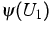can be a disc of an arbitrarily small radius with its center located anywhere. Thus, the class of maps f contains information about the behavior of arbitrarily long iterations of f on arbitrarily fine spatial scales.

The general intuition here is that if the class of maps is large, then the dynamics is rich, while if the dynamics is sufficiently simple, then the class is somehow restricted. The following definition introduces maps whose dynamics is ultimately rich.

Definition. A Cr-smooth symplectic map f is called universal (or Cr-universal) if its dynamical class is dense in the Cr-topology among all Cr-smooth symplectic diffeomorphisms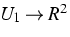.

By this definition, the detailed understanding of the dynamics of any single universal map is not simpler than the understanding of all other area-preserving maps altogether, i.e., it is beyond human abilities. What is astonishing, is that such universal maps are quite common. Namely, we show in  that the following statement is valid.

Theorem 3. Cr-universal maps exist in any neighborhood (in the Cr-topology) of any area-preserving map with a homoclinic tangency, i.e., they are dense in the Newhouse regions.

This theorem says that universal maps occur as often as homoclinic tangencies do. It is an empirical fact that if we have any explicitly given area-preserving map with chaotic behavior, then by slightly changing parameters we can quite often encounter a homoclinic tangency. Thus, according to the theorem above, if we have enough control parameters then we can tune our map as close as we want to any other area-preserving map in some carefully chosen coordinates.

#### Project 3: Problems of synchronization

Cooperation with: L.P. Shilnikov (Institute for Applied Mathematics and Cybernetics, Nizhny Novgorod, Russia)

Supported by: Prize of the Alexander von Humboldt Foundation

Description: The problem of synchronization is one of the main problems of applied signal processing. It can be formulated as follows: Let a generator of periodic oscillations be forced by an external periodic signal. Under which conditions will the generator respond with the same frequency as the external signal? Can it respond to the signal with a fractional or multiple frequency? Which response should we expect in general?

When the amplitude of the external force is small, these questions are solved by the classical method of averaging: depending on the frequency detuning, either synchronization or beating regimes should be expected. In the case of finite amplitude, the situation becomes much more complicated: synchronization of different frequencies is possible for the same parameter values, as well as chaotic regimes. In  we study the problem of sudden transition from synchronization to chaos. This process can be modeled in geometrical terms as a bifurcation of a saddle-node periodic orbit with a non-smooth homoclinic loop (see Figure 1). We give criteria which allow to distinguish between two possible scenarios of what happens when the saddle-node has disappeared: either the dynamics is always chaotic, or zones of chaotic behavior interchange in the parameter space with zones of synchronization.

We also analyze in  the problem of coexistence of chaotic and synchronized behavior. The classical result of Cartwright and Littlewood from 1945  (explaining earlier experiments by van der Pol) is that the coexistence of two synchronization regimes with different frequencies implies also the existence of a chaotic transient. We enhanced this by giving criteria for the coexistence of chaos with a single synchronized regime and for the existence of chaos in the absence of stable synchronized regimes.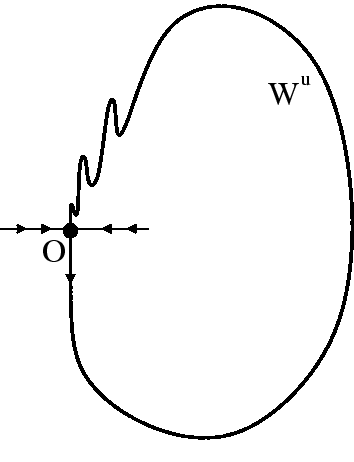References:

1. N.K. GAVRILOV, L.P. SHILNIKOV, Three-dimensional dynamical systems close to systems with a structurally unstable homoclinic curve, II, Math. USSR-Sb., 19 (1973), pp. 139-156.
2. S.V. GONCHENKO, L.P. SHILNIKOV, D.V. TURAEV, On models with non-rough Poincaré homoclinic curves, Physica D, 62 (1993), pp. 1-14.
3. D. TURAEV, On dimension of non-local bifurcational problems, Bifurcation and Chaos, 6 (1996), pp.123-156.
4. S.V. GONCHENKO, L.P. SHILNIKOV, D.V. TURAEV, On dynamical properties of diffeomorphisms with homoclinic tangencies, WIAS Preprint no. 795 , 2002 (to be submitted).
5. D. TURAEV, Polynomial approximations of symplectic dynamics and richness of chaos in non-hyperbolic area-preserving maps, Nonlinearity, 16 (2003), pp. 1-13.
6. L.P. SHILNIKOV, D.V. TURAEV, Some mathematical problems of classical synchronization, to appear in: Mathematics. Mechanics. Informatics. 10 years of Russian Foundation of Basic Research, Moscow, 2003.
7. M.L. CARTWRIGHT, J.E. LITTLEWOOD, On nonlinear differential equations of the second order: I. The equation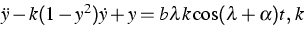large, J. London Math. Soc., 20 (1945), pp. 180-189.

 [Next]: Research Group Numerical Mathematics and Scientific
 [Up]: Project descriptions
 [Previous]: Multiscale systems
[Contents]   [Index]

LaTeX typesetting by I. Bremer
5/16/2003